Test: Power Electronics- 5

# Test: Power Electronics- 5 - Electrical Engineering (EE)

Test Description

## 10 Questions MCQ Test GATE Electrical Engineering (EE) 2023 Mock Test Series - Test: Power Electronics- 5

Test: Power Electronics- 5 for Electrical Engineering (EE) 2023 is part of GATE Electrical Engineering (EE) 2023 Mock Test Series preparation. The Test: Power Electronics- 5 questions and answers have been prepared according to the Electrical Engineering (EE) exam syllabus.The Test: Power Electronics- 5 MCQs are made for Electrical Engineering (EE) 2023 Exam. Find important definitions, questions, notes, meanings, examples, exercises, MCQs and online tests for Test: Power Electronics- 5 below.
Solutions of Test: Power Electronics- 5 questions in English are available as part of our GATE Electrical Engineering (EE) 2023 Mock Test Series for Electrical Engineering (EE) & Test: Power Electronics- 5 solutions in Hindi for GATE Electrical Engineering (EE) 2023 Mock Test Series course. Download more important topics, notes, lectures and mock test series for Electrical Engineering (EE) Exam by signing up for free. Attempt Test: Power Electronics- 5 | 10 questions in 30 minutes | Mock test for Electrical Engineering (EE) preparation | Free important questions MCQ to study GATE Electrical Engineering (EE) 2023 Mock Test Series for Electrical Engineering (EE) Exam | Download free PDF with solutions
 1 Crore+ students have signed up on EduRev. Have you?
*Answer can only contain numeric values
Test: Power Electronics- 5 - Question 1

### A 3 ϕ 180°-mode bridge inverter has star-connected load of R = 8 Ω and L-30 mH. The inverter is fed from 220 V dc and its output frequency is 50 Hz. Find the RMS value of 3rd harmonic of line current (in A)

Detailed Solution for Test: Power Electronics- 5 - Question 1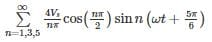All triplen harmonics are absent from the line voltage

*Answer can only contain numeric values
Test: Power Electronics- 5 - Question 2

### Find the commutation time (in ms) for a 3 phase full converter (RLE load) at firing angle 30°. (Answer should be in ms)

Detailed Solution for Test: Power Electronics- 5 - Question 2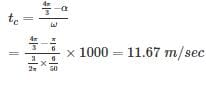Test: Power Electronics- 5 - Question 3

### A 6-pulse thyristor bridge is connected to a balanced 50 Hz , 3 - ϕ ac source. Assuming the dc o/p current of the rectifier is constant, the lowest frequency harmonic component of the source line current is

Detailed Solution for Test: Power Electronics- 5 - Question 3

Ripple frequency = 5 x 50 = 250 Hz. Hence the least frequency component is of 250 Hz

Test: Power Electronics- 5 - Question 4

The overlap angle of a phase controlled converter will increase if

1. The firing angle increases
2. The frequency of supply increases
3. The supply voltage increases

Among the above statements

Detailed Solution for Test: Power Electronics- 5 - Question 4

With increase in frequency the effective reactance at source increases which increase overlap angle. If supply voltage increases current will take more time to build up through source inductance, hence μ increases.  If firing angle increases to maintain constant average current overlap angle will decrease. (observe by putting α=0,30,60 etc)

Test: Power Electronics- 5 - Question 5

A separately excited DC motor fed from 3-phase semi converter develop a full load torque at 1500 rpm when firing angle is 60°. The armature taking 50 A at 400 V DC and having the resistance of 0.4 Ω, then supply voltage per phase will be ________

Detailed Solution for Test: Power Electronics- 5 - Question 5

Concept: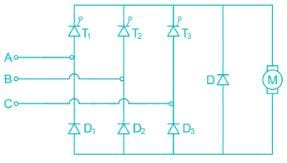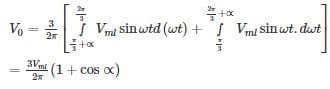Calculation:

We know that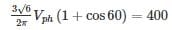VPh = 228 V

*Answer can only contain numeric values
Test: Power Electronics- 5 - Question 6

A 3 ϕ 180°-mode bridge inverter has star-connected load of R = 8 Ω and L-30 mH. The inverter is fed from 220 V dc and its output frequency is 50 Hz. Find the R.M.S value of 1st harmonic current (in A)

Detailed Solution for Test: Power Electronics- 5 - Question 6

Vs = 220 V, R = 8 Ω, ωL = 2π × 50 × 30 × 10-3 = 9.42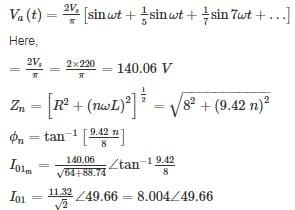*Answer can only contain numeric values
Test: Power Electronics- 5 - Question 7

A 3-ϕ, 3 pulse converter, fed from delta star transformer, is connected to a load requiring ripple free current. A freewheeling diode is connected across the load. Find the R.M.S value of thyristor current (in A) for firing angle 60°. (Take Vmp = 200 V)

Detailed Solution for Test: Power Electronics- 5 - Question 7

Average value of the output voltage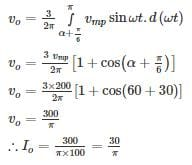R.M.S value of thyristor current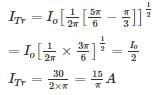Test: Power Electronics- 5 - Question 8

A 240 V battery is being charged through a three phase diode bridge rectifier. The rectifier is fed by a three – phase 230 V. 50 Hz source current limiting resistance in series with battery is 8Ω and load current is assumed to be ripple free. The value of average battery charge current is –

Detailed Solution for Test: Power Electronics- 5 - Question 8

Vm = √2 × 230 V
Average output voltage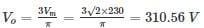let the battery emf is E and average charging current is Io then,
VO = E + IO R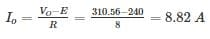*Answer can only contain numeric values
Test: Power Electronics- 5 - Question 9

A solar cell of 400 V is feeding power to an ac supply of 440 V, 50 Hz through a 3 – phase fully controlled bridge converter. A large inductance is connected in the dc circuit to maintain the dc current at 12 A. If the solar cell resistance is 1 Ω, then each thyristor will be reverse biased for a period of – (in degrees)

Detailed Solution for Test: Power Electronics- 5 - Question 9

Rsolar = 1 Ω, Io = 12 A

Vs = 400 – 12 (1) = 388 V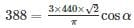⇒ α = 49.23°

So each thyristor will reverse biased for

180° - 49.23 = 130.77°

Test: Power Electronics- 5 - Question 10

A three phase fully controlled bridge converter is feeding a load drawing a constant and ripple free load current of 20 A at a firing angle of 60°. The approximate total harmonic distortion (% THD) and the rms value of fundamental component of input current will respectively

Detailed Solution for Test: Power Electronics- 5 - Question 10

Total rms current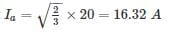Fundamental current Ia1 = 0.78 × 20 = 15.6A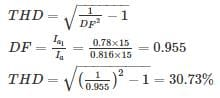## GATE Electrical Engineering (EE) 2023 Mock Test Series

22 docs|284 tests
Information about Test: Power Electronics- 5 Page
In this test you can find the Exam questions for Test: Power Electronics- 5 solved & explained in the simplest way possible. Besides giving Questions and answers for Test: Power Electronics- 5, EduRev gives you an ample number of Online tests for practice

## GATE Electrical Engineering (EE) 2023 Mock Test Series

22 docs|284 tests(Scan QR code)